# Petrov classification

(diff) ← Older revision | Latest revision (diff) | Newer revision → (diff)

The space-time of general relativity theory consists of a connected Hausdorff manifold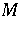of dimension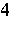, called the space-time, which admits a metric tensor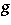of Lorentz signature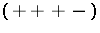. A tensor of particular importance from both the physical and mathematical viewpoint is the Weyl tensor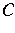. This tensor has the property that if the metricis replaced by a metric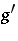which is conformally related to it (so thatfor a nowhere-zero real-valued function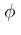on), then it is unchanged. Ifis zero on, thenis locally conformally related to a flat metric on. The tensoris given in components in terms of the curvature components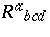, the Ricci tensor components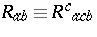, and the Ricci scalar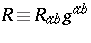(and with square brackets denoting the usual skew-symmetrization of indices) by(a1)

In the late 1940s and early 1950s, A.Z. Petrov developed some elegant algebraic techniques which led to the classification of the Weyl tensor and which now bears his name [a1]. Essentially, one takes the Weyl tensor with components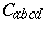and, making use of the symmetries of this tensor, writes them in "block index" form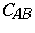where each capital index can take a value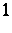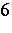and represents a pair of skew indices according to the scheme:represents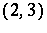,represents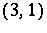,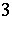represents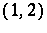,represents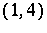,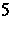represents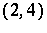,represents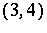. Thus,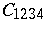becomes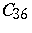, etc. Then one converts the resulting-matrix to a complex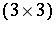-matrix which, in a well-defined sense, is equivalent to it and which, by the symmetries of the Weyl tensor, is symmetric and trace-free. One then proceeds to classify the Weyl tensor by the Jordan form of this matrix which, from the trace-free condition, must either be (in Segre's notation; see Segre classification),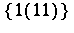,,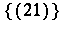,, or the zero matrix at a point of(the type being dependent on the point!). The trace-free condition shows that in the last three types all eigenvalues are zero. These possibilities are the Petrov types and they are conventionally labelled I, D, II, N, III, and 0, respectively. If a space-time has the same Petrov type at each point it is said to be of that type. A space-time of type 0 is conformally flat. For a vacuum space-time (one for which the Ricci tensor is zero on) the Riemann tensor and the Weyl tensor are equal and so the Petrov classification applies to the Riemann tensor. Although it is more convenient to "go complex" to achieve these results, it is not necessary. One can work entirely in the field of real numbers, but the loss of algebraic closure means that one must deal with the case of complex eigenvalues as well as the (real) Jordan forms.

In the 1960s much further work was done on the Petrov classification, see [a2], [a3], [a4], [a5]. Associated with each Petrov type at at point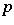inare"principal" null directions (some possibly coincident). These directions are instrumental in the physical interpretation of the Petrov type and there are four distinct ones associated with type I, two with type D (both repeated), three with type II (one repeated), two with type III (one triply repeated), and one with type N (quadruply repeated). These directions have a rather elegant relationship with the Weyl tensor and were explored in detail by L. Bel and R.K. Sachs. R. Penrose was able to give a simpler approach to them using spinors instead of tensors [a4].

One of the early uses of the Petrov classification was in the finding of exact solutions of the Einstein equations [a6]. The idea was that one looked for space-times of a particular Petrov type (together with other restrictions) to simplify the situation. The Petrov classification also fits elegantly into those powerful calculatory techniques usually referred to as the Newman–Penrose formalism [a7].

How to Cite This Entry:
Petrov classification. Encyclopedia of Mathematics. URL: http://encyclopediaofmath.org/index.php?title=Petrov_classification&oldid=15004
This article was adapted from an original article by G.S. Hall (originator), which appeared in Encyclopedia of Mathematics - ISBN 1402006098. See original article